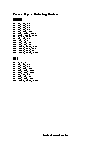Receive free math worksheets via email:

Multiplying Ones!
1 x 1 = 1
11 x 11 = 121
111 x 111 = 12321
1111 x 1111 = 1234321
11111 x 11111 = 123454321# Ordering Numbers Worksheets= free= members onlyBasic Lesson
Students learn the concept of sequence by greatest to least and vice versa.
Standard: MATH 1View worksheetIntermediate Lesson
Students practice ordering very large numbers.
Standard: MATH 1View worksheetIndependent Practice 1
20 problems for students order numbers from least to greatest.
Standard: MATH 1View worksheetIndependent Practice 2
Order numbers from least to greatest to complete each problem.
Standard: MATH 1View worksheetHomework Worksheet
16 Ordering Numbers problems with a completed example.
Standard: MATH 1View worksheetOrdering Numbers Quiz
Provides a mixture of ordering numbers problems.
Standard: MATH 1View worksheetAnswers for the homework and quiz.View answersAnswers for both lessons and both practice sheets.View answersUnderstanding Patterns and Sequences in the Stock Market
Understanding patterns and sequences in the stock market helps you to determine the current demand and supply forces in the stock market.View Math ArticleWant to be better at Video Games? Learn more about Patterns!
You need to be able to recognize patterns in the game. Video games are all based on patterns.View Math Article